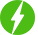# Logistic Regression in Python – Credit Default Prediction

2

Logistic Regression in Python – Credit Default Prediction, Learn key components of logistic regression and develop a logistic regression model using python.

## Course Description

There are different types of statistical, data mining and machine learning algorithms in Predictive Modeling. Each algorithm is used to address the specific needs of the business concern. So choosing the right algorithm for your business is a great task. Regression algorithm is one among them. Regression algorithm is used to forecast continuous data like credit scoring or predicting the next outcome of a time based event. For example regression algorithm can be used to predict the trend of a stock movement with its past prices.

Regression is a statistical method which helps to determine the relationship between one dependent variable and other independent variables. It explains how the dependent variable changes when one of the independent variable varies. It is also used to know which independent variable is related to the dependent variable and what is their relationship. Regression analysis is widely used in the field of prediction and forecasting. Regression analysis is an important component for modelling and analyzing data.

In the recent years many techniques have been developed to perform regression analysis. They are Linear regression, Logistic regression, Polynomial regression, Stepwise regression, Ridge regression, Lasso Regression and Elastic net regression.

Logistic regression is also known as logit regression or logit model. This is used to find the probability of event success and event failure. Logistic regression determines the relationship between categorical dependent variable and one or more independent variables using a logistic function.

Logistic regression is used for predicting the probability of occurrence of an event by fitting the data to a logistic curve. Ordinary Least Squares on the other hand is an important computational problem that is used in applications when there is a need to use a linear mathematical model to measurements which are derived from the experiments. OLS takes various forms like Correlation, multiple regression, ANOVA and others. Logistic regression is most widely used in the field of medical science whereas OLS is mostly used in social sciences.

Free \$54.99
We will be happy to hear your thoughtsRegister New Account
• Total (0)
0